# Electronics and Communication Engineering - Networks Analysis and Synthesis

6.

When a current source I is suddenly connected across a two terminal relaxed RC circuit at time t = 0, the voltage across the current source is shown in figure. The RC circuit is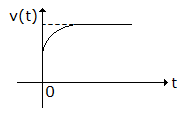A. a series combination of R and C B. a parallel combination of R and C C. a series combination of R and parallel combination of R and C D. a pure capacitor

Explanation:

Initially capacitor behaves as short-circuit and finally as open circuit.

7.

The driving-point impedance Z(s) of a network has a pole-zero locations as shown in the figure. If Z(0) = 3, then Z (s) is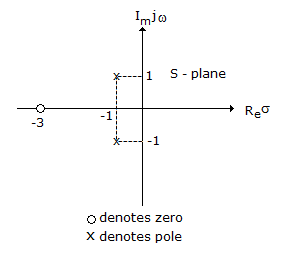A.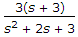B.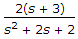C.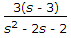D.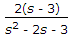Explanation:

From figure, The impedance function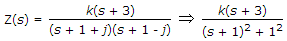and Z(0) =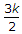while in question Z(0) = 3= 3k = 2.

8.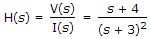and i(t) is a unit step, then V(t) in the steady state is given by

 A. 4/9 B. 4/3 C. 0 D. ∞

Explanation: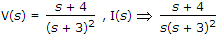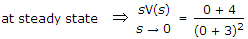= 4/9.

9.

For a transmission line open circuit and short circuit impedances are 20 Ω and 5 Ω. Then characteristic impedance is

 A. 100 Ω B. 50 Ω C. 25 Ω D. 10 Ω

Explanation:

Z0 = Zoc Zsc .

10.

The terms RMS and average values apply only to sine waves.

 A. True B. False